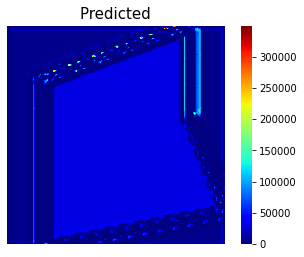# Creating custom loss function in CNN for regression

I am trying to make my CNN regression network work, but output is far from ground truth. The input is a binary image (600x600) which the background is black, and foreground is white. The ground truth associated with each input is an image with color range from 0 t 255 which is normalized between 0 and 1.

Below, you can see one sample of Input (x), ground truth(y) and predicted output (y_hat), respectively. The network can almost detect edges and background but in foreground all the predicted values are almost same (see predicted one).

I believe that since all foregrounds in input are 1, network cannot update weights correctly and it may need custom loss function to penalize loss function.

I am wondering whether my idea is right or not, if yes what kind of custom function I need?

I tried to penalize the foreground by custom loss function below, but it didn’t improve the result.

mse = nn.MSELoss(reduction=‘mean’)

def criterion(y, y_hat, x, loss, weight=0.1):
y_hat_modified = torch.where(x[:,0:1]==1, weight*y_hat,y_hat) # x[:,0:1] is input
return loss(y,y_hat_modified)